xSurface integralOverview

In mathematics
Mathematics
Mathematics is the study of quantity, space, structure, and change. Mathematicians seek out patterns and formulate new conjectures. Mathematicians resolve the truth or falsity of conjectures by mathematical proofs, which are arguments sufficient to convince other mathematicians of their validity...

, a surface integral is a definite integral taken over a surface
Surface
In mathematics, specifically in topology, a surface is a two-dimensional topological manifold. The most familiar examples are those that arise as the boundaries of solid objects in ordinary three-dimensional Euclidean space R3 — for example, the surface of a ball...

(which may be a curve set in space
Space
Space is the boundless, three-dimensional extent in which objects and events occur and have relative position and direction. Physical space is often conceived in three linear dimensions, although modern physicists usually consider it, with time, to be part of a boundless four-dimensional continuum...

); it can be thought of as the double integral analog of the line integral
Line integral
In mathematics, a line integral is an integral where the function to be integrated is evaluated along a curve.The function to be integrated may be a scalar field or a vector field...

. Given a surface, one may integrate over its scalar field
Scalar field
In mathematics and physics, a scalar field associates a scalar value to every point in a space. The scalar may either be a mathematical number, or a physical quantity. Scalar fields are required to be coordinate-independent, meaning that any two observers using the same units will agree on the...

s (that is, function
Function (mathematics)
In mathematics, a function associates one quantity, the argument of the function, also known as the input, with another quantity, the value of the function, also known as the output. A function assigns exactly one output to each input. The argument and the value may be real numbers, but they can...

s which return number
Number
A number is a mathematical object used to count and measure. In mathematics, the definition of number has been extended over the years to include such numbers as zero, negative numbers, rational numbers, irrational numbers, and complex numbers....

s as values), and vector field
Vector field
In vector calculus, a vector field is an assignmentof a vector to each point in a subset of Euclidean space. A vector field in the plane for instance can be visualized as an arrow, with a given magnitude and direction, attached to each point in the plane...

s (that is, functions which return vectors as values).

Surface integrals have applications in physics
Physics
Physics is a natural science that involves the study of matter and its motion through spacetime, along with related concepts such as energy and force. More broadly, it is the general analysis of nature, conducted in order to understand how the universe behaves.Physics is one of the oldest academic...

, particularly with the classical theory of electromagnetism
Electromagnetism
Electromagnetism is one of the four fundamental interactions in nature. The other three are the strong interaction, the weak interaction and gravitation...

.
Consider a surface S on which a scalar field f is defined.Encyclopedia
In mathematics
Mathematics
Mathematics is the study of quantity, space, structure, and change. Mathematicians seek out patterns and formulate new conjectures. Mathematicians resolve the truth or falsity of conjectures by mathematical proofs, which are arguments sufficient to convince other mathematicians of their validity...

, a surface integral is a definite integral taken over a surface
Surface
In mathematics, specifically in topology, a surface is a two-dimensional topological manifold. The most familiar examples are those that arise as the boundaries of solid objects in ordinary three-dimensional Euclidean space R3 — for example, the surface of a ball...

(which may be a curve set in space
Space
Space is the boundless, three-dimensional extent in which objects and events occur and have relative position and direction. Physical space is often conceived in three linear dimensions, although modern physicists usually consider it, with time, to be part of a boundless four-dimensional continuum...

); it can be thought of as the double integral analog of the line integral
Line integral
In mathematics, a line integral is an integral where the function to be integrated is evaluated along a curve.The function to be integrated may be a scalar field or a vector field...

. Given a surface, one may integrate over its scalar field
Scalar field
In mathematics and physics, a scalar field associates a scalar value to every point in a space. The scalar may either be a mathematical number, or a physical quantity. Scalar fields are required to be coordinate-independent, meaning that any two observers using the same units will agree on the...

s (that is, function
Function (mathematics)
In mathematics, a function associates one quantity, the argument of the function, also known as the input, with another quantity, the value of the function, also known as the output. A function assigns exactly one output to each input. The argument and the value may be real numbers, but they can...

s which return number
Number
A number is a mathematical object used to count and measure. In mathematics, the definition of number has been extended over the years to include such numbers as zero, negative numbers, rational numbers, irrational numbers, and complex numbers....

s as values), and vector field
Vector field
In vector calculus, a vector field is an assignmentof a vector to each point in a subset of Euclidean space. A vector field in the plane for instance can be visualized as an arrow, with a given magnitude and direction, attached to each point in the plane...

s (that is, functions which return vectors as values).

Surface integrals have applications in physics
Physics
Physics is a natural science that involves the study of matter and its motion through spacetime, along with related concepts such as energy and force. More broadly, it is the general analysis of nature, conducted in order to understand how the universe behaves.Physics is one of the oldest academic...

, particularly with the classical theory of electromagnetism
Electromagnetism
Electromagnetism is one of the four fundamental interactions in nature. The other three are the strong interaction, the weak interaction and gravitation...

.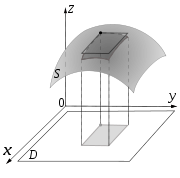## Surface integrals of scalar fields

Consider a surface S on which a scalar field f is defined. If we think of S as made of some material, and for each x in S the number f(x) is the density
Density
The mass density or density of a material is defined as its mass per unit volume. The symbol most often used for density is ρ . In some cases , density is also defined as its weight per unit volume; although, this quantity is more properly called specific weight...

of material at x, then the surface integral of f over S is the mass
Mass
Mass can be defined as a quantitive measure of the resistance an object has to change in its velocity.In physics, mass commonly refers to any of the following three properties of matter, which have been shown experimentally to be equivalent:...

per unit thickness of S. (This only holds as long as the surface is an infinitesimally thin shell.) One approach to calculate the surface integral is then to split the surface in many very small pieces, assume that on each piece the density is approximately constant, find the mass per unit thickness of each piece by multiplying the density of the piece by its area, and then sum up the resulting numbers to find the total mass per unit thickness of S.

To find an explicit formula for the surface integral, we need to parameterize
Coordinate system
In geometry, a coordinate system is a system which uses one or more numbers, or coordinates, to uniquely determine the position of a point or other geometric element. The order of the coordinates is significant and they are sometimes identified by their position in an ordered tuple and sometimes by...

S by considering on S a system of curvilinear coordinates
Curvilinear coordinates
Curvilinear coordinates are a coordinate system for Euclidean space in which the coordinate lines may be curved. These coordinates may be derived from a set of Cartesian coordinates by using a transformation that is locally invertible at each point. This means that one can convert a point given...

, like the latitude and longitude
Geographic coordinate system
A geographic coordinate system is a coordinate system that enables every location on the Earth to be specified by a set of numbers. The coordinates are often chosen such that one of the numbers represent vertical position, and two or three of the numbers represent horizontal position...

on a sphere
Sphere
A sphere is a perfectly round geometrical object in three-dimensional space, such as the shape of a round ball. Like a circle in two dimensions, a perfect sphere is completely symmetrical around its center, with all points on the surface lying the same distance r from the center point...

. Let such a parameterization be x(s, t), where (s, t) varies in some region T in the plane. Then, the surface integral is given by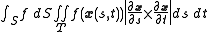where the expression between bars on the right-hand side is the magnitude
Magnitude (mathematics)
The magnitude of an object in mathematics is its size: a property by which it can be compared as larger or smaller than other objects of the same kind; in technical terms, an ordering of the class of objects to which it belongs....

of the cross product
Cross product
In mathematics, the cross product, vector product, or Gibbs vector product is a binary operation on two vectors in three-dimensional space. It results in a vector which is perpendicular to both of the vectors being multiplied and normal to the plane containing them...

of the partial derivative
Partial derivative
In mathematics, a partial derivative of a function of several variables is its derivative with respect to one of those variables, with the others held constant...

s of x(s, t).

For example, if we want to find the surface area of some general functional shape, say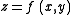, we have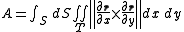where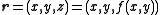. So that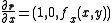, and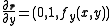. So,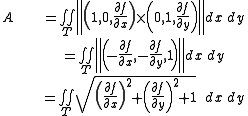which is the familiar formula we get for the surface area of a general functional shape. One can recognize the vector in the second line above as the normal vector
Surface normal
A surface normal, or simply normal, to a flat surface is a vector that is perpendicular to that surface. A normal to a non-flat surface at a point P on the surface is a vector perpendicular to the tangent plane to that surface at P. The word "normal" is also used as an adjective: a line normal to a...

to the surface.

Note that because of the presence of the cross product, the above formulas only work for surfaces embedded in three dimensional space.

## Surface integrals of vector fields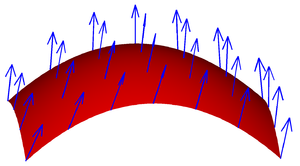Consider a vector field v on S, that is, for each x in S, v(x) is a vector.

The surface integral can be defined component-wise according to the definition of the surface integral of a scalar field; the result is a vector. This applies for example in the expression of the electric field at some fixed point due to an electrically charged surface, or the gravity at some fixed point due to a sheet of material.

Alternatively we integrate the normal component of the vector field; the result is a scalar. Imagine that we have a fluid flowing through S, such that v(x) determines the velocity of the fluid at x. The flux
Flux
In the various subfields of physics, there exist two common usages of the term flux, both with rigorous mathematical frameworks.* In the study of transport phenomena , flux is defined as flow per unit area, where flow is the movement of some quantity per time...

is defined as the quantity of fluid flowing through S in unit amount of time.

This illustration implies that if the vector field is tangent
Tangent
In geometry, the tangent line to a plane curve at a given point is the straight line that "just touches" the curve at that point. More precisely, a straight line is said to be a tangent of a curve at a point on the curve if the line passes through the point on the curve and has slope where f...

to S at each point, then the flux is zero, because the fluid just flows in parallel
Parallel (geometry)
Parallelism is a term in geometry and in everyday life that refers to a property in Euclidean space of two or more lines or planes, or a combination of these. The assumed existence and properties of parallel lines are the basis of Euclid's parallel postulate. Two lines in a plane that do not...

to S, and neither in nor out. This also implies that if v does not just flow along S, that is, if v has both a tangential and a normal component, then only the normal component contributes to the flux. Based on this reasoning, to find the flux, we need to take the dot product
Dot product
In mathematics, the dot product or scalar product is an algebraic operation that takes two equal-length sequences of numbers and returns a single number obtained by multiplying corresponding entries and then summing those products...

of v with the unit surface normal
Surface normal
A surface normal, or simply normal, to a flat surface is a vector that is perpendicular to that surface. A normal to a non-flat surface at a point P on the surface is a vector perpendicular to the tangent plane to that surface at P. The word "normal" is also used as an adjective: a line normal to a...

to S at each point, which will give us a scalar field, and integrate the obtained field as above. We find the formula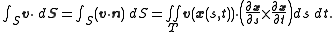The cross product on the right-hand side of this expression is a surface normal determined by the parametrization.

This formula defines the integral on the left (note the dot and the vector notation for the surface element).

## Surface integrals of differential 2-forms

Let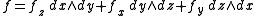be a differential 2-form
Differential form
In the mathematical fields of differential geometry and tensor calculus, differential forms are an approach to multivariable calculus that is independent of coordinates. Differential forms provide a better definition for integrands in calculus...

defined on the surface S, and let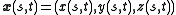be an orientation preserving
Orientability
In mathematics, orientability is a property of surfaces in Euclidean space measuring whether or not it is possible to make a consistent choice of surface normal vector at every point. A choice of surface normal allows one to use the right-hand rule to define a "clockwise" direction of loops in the...

parametrization of S with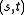in D. Then, the surface integral of f on S is given by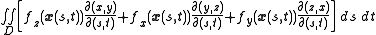where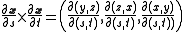is the surface element normal to S.

Let us note that the surface integral of this 2-form is the same as the surface integral of the vector field which has as components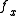,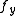and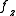.

## Theorems involving surface integrals

Various useful results for surface integrals can be derived using differential geometry and vector calculus, such as the divergence theorem
Divergence theorem
In vector calculus, the divergence theorem, also known as Gauss' theorem , Ostrogradsky's theorem , or Gauss–Ostrogradsky theorem is a result that relates the flow of a vector field through a surface to the behavior of the vector field inside the surface.More precisely, the divergence theorem...

, and its generalization, Stokes' theorem
Stokes' theorem
In differential geometry, Stokes' theorem is a statement about the integration of differential forms on manifolds, which both simplifies and generalizes several theorems from vector calculus. Lord Kelvin first discovered the result and communicated it to George Stokes in July 1850...

.

Let us notice that we defined the surface integral by using a parametrization of the surface S. We know that a given surface might have several parametrizations. For example, if we move the locations of the North Pole and South Pole on a sphere, the latitude and longitude change for all the points on the sphere. A natural question is then whether the definition of the surface integral depends on the chosen parametrization. For integrals of scalar fields, the answer to this question is simple, the value of the surface integral will be the same no matter what parametrization one uses.

For integrals of vector fields things are more complicated, because the surface normal is involved. It can be proved that given two parametrizations of the same surface, whose surface normals point in the same direction, one obtains the same value for the surface integral with both parametrizations. If, however, the normals for these parametrizations point in opposite directions, the value of the surface integral obtained using one parametrization is the negative of the one obtained via the other parametrization. It follows that given a surface, we do not need to stick to any unique parametrization; but, when integrating vector fields, we do need to decide in advance which direction the normal will point to and then choose any parametrization consistent with that direction.

Another issue is that sometimes surfaces do not have parametrizations which cover the whole surface; this is true for example for the surface of a cylinder
Cylinder (geometry)
A cylinder is one of the most basic curvilinear geometric shapes, the surface formed by the points at a fixed distance from a given line segment, the axis of the cylinder. The solid enclosed by this surface and by two planes perpendicular to the axis is also called a cylinder...

(of finite height). The obvious solution is then to split that surface in several pieces, calculate the surface integral on each piece, and then add them all up. This is indeed how things work, but when integrating vector fields one needs to again be careful how to choose the normal-pointing vector for each piece of the surface, so that when the pieces are put back together, the results are consistent. For the cylinder, this means that if we decide that for the side region the normal will point out of the body, then for the top and bottom circular parts the normal must point out of the body too.

Lastly, there are surfaces which do not admit a surface normal at each point with consistent results (for example, the Möbius strip
Möbius strip
The Möbius strip or Möbius band is a surface with only one side and only one boundary component. The Möbius strip has the mathematical property of being non-orientable. It can be realized as a ruled surface...

). If such a surface is split into pieces, on each piece a parametrization and corresponding surface normal is chosen, and the pieces are put back together, we will find that the normal vectors coming from different pieces cannot be reconciled. This means that at some junction between two pieces we will have normal vectors pointing in opposite directions. Such a surface is called non-orientable
Orientability
In mathematics, orientability is a property of surfaces in Euclidean space measuring whether or not it is possible to make a consistent choice of surface normal vector at every point. A choice of surface normal allows one to use the right-hand rule to define a "clockwise" direction of loops in the...

, and on this kind of surface one cannot talk about integrating vector fields.

• Divergence theorem
Divergence theorem
In vector calculus, the divergence theorem, also known as Gauss' theorem , Ostrogradsky's theorem , or Gauss–Ostrogradsky theorem is a result that relates the flow of a vector field through a surface to the behavior of the vector field inside the surface.More precisely, the divergence theorem...

• Stokes' theorem
Stokes' theorem
In differential geometry, Stokes' theorem is a statement about the integration of differential forms on manifolds, which both simplifies and generalizes several theorems from vector calculus. Lord Kelvin first discovered the result and communicated it to George Stokes in July 1850...

• Line integral
Line integral
In mathematics, a line integral is an integral where the function to be integrated is evaluated along a curve.The function to be integrated may be a scalar field or a vector field...

• Volume integral
Volume integral
In mathematics — in particular, in multivariable calculus — a volume integral refers to an integral over a 3-dimensional domain....

• Cartesian Coordinate System
Cartesian coordinate system
A Cartesian coordinate system specifies each point uniquely in a plane by a pair of numerical coordinates, which are the signed distances from the point to two fixed perpendicular directed lines, measured in the same unit of length...

• Volume and Surface Area Elements in Spherical Coordinate Systems
• Volume and Surface Area Elements in Cylindrical Coordinate Systems
• Holstein–Herring method